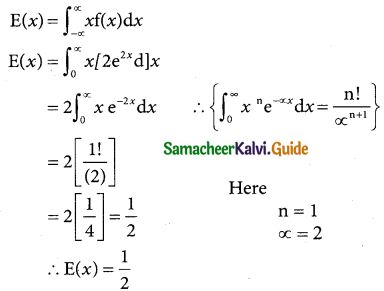Tamilnadu State Board New Syllabus Samacheer Kalvi 12th Business Maths Guide Pdf Chapter 6 Random Variable and Mathematical Expectation Miscellaneous Problems Text Book Back Questions and Answers, Notes.

## Tamilnadu Samacheer Kalvi 12th Business Maths Solutions Chapter 6 Random Variable and Mathematical Expectation Miscellaneous Problems

Question 1.
The probability function of a random variable X is given by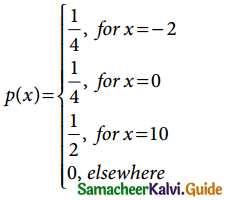Evaluate the following probabilities.
(i) P(X ≤ 0)
(ii) P(X ≤ 0)
(iii) P( X ≤ 2) and
(iv) P(0 ≤ X ≤ 10)
Solution:
(i) From the data

 x -2 0 10 P(x = x) 1/4 1/4 1/2

(i) P(X ≤ 0) = P (X = 0) + P (X = -2)
$$=\frac{1}{4}+\frac{1}{4}=\frac{1}{2}$$
(ii) P(X < 0) = P (X = – 2) = $$\frac{1}{4}$$
(iii) P(|X| ≤ 2) = P(-2 ≤ X ≤ 2)
= P(X = -2) + P(X = -1) + P(X = 0) + P(X = 1) + P(X = 2)
= $$\frac{1}{4}$$ + 0 + $$\frac{1}{4}$$ + 0 + 0
= $$\frac{1}{2}$$
(iv) P(0 ≤ X ≤ 10) = P(X = 0) + P(X = 10) + 0
$$=\frac{1}{4}+\frac{1}{2}=\frac{3}{4}$$Question 2.
The probability function of a random variable X is given by(a) Compute: (i) P(1 ≤ X ≤ 2) and (ii) P(X = 3).
Solution:
W.K.T Probability density Function
f(x) = $$\frac { d[F(x)] }{dx}$$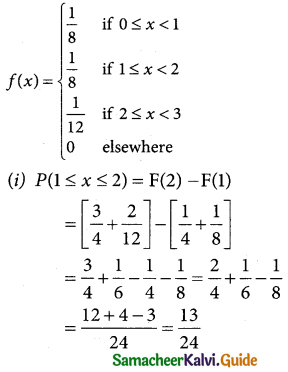(ii) P(X = 3) = 0
(b) X is not discrete since f is not a step function.Question 3.
The p.d.f. of X is defined as
f(x) = $$\left\{\begin{array}{l} \mathrm{k}, \text { for } 0<x \leq 4 \\ 0, \text { otherwise } \end{array}\right.$$
Solution:
Let X and a random variable if a Probability density function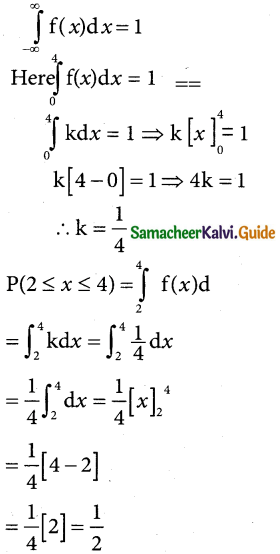Question 4.
The probability distribution function of a discrete random variable X is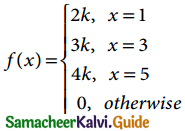where k is some constant. Find (a) k and (b) P(X > 2).
Solution:
(a) Let X be the random variable of a probability distribution function
W.K.T Σpi = 1
P(x = 1) + P(x = 3) + P(x = 5) = 1
2k + 3k + 4k = 1
9k – 1 ⇒ k = 1/9

(b) P(x > 1) = P(x = 3) + P(x = 5)
= 3k + 4k = 7k
= 7(1/9) = 7/9Question 5.
The probability distribution function of a discrete random variable X is
f(x) = $$\left\{\begin{array}{l} \mathrm{a}+\mathrm{b} x^{2}, 0 \leq x \leq 1 \\ 0, \text { otherwise } \end{array}\right.$$
where a and b are some constants. Find (i) a and b if E(X) = 3/5 (ii) Var(X).
Solution:
Let X be due to continuous variable of the density function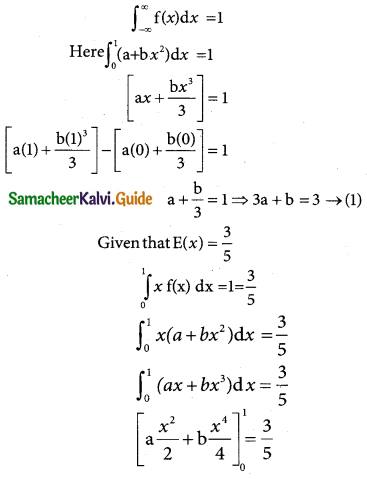Question 6.
Prove that if E(X) = 0, then V(X) = E(X²)
Solution:
Given E(X) = 0. To show V(X) = E (X2)
We know that Var (X) = E(X2) – [E(X)]2
So if E(X) = 0, Var (X) = E(X2)
From the definition of the variance of X also we can see the result.
Var(X) = Σ[x – E(x)]2 p(x)
If E (X) = 0, then V(X) = Σ x2 p(x) = E(X2)Question 7.
What is the expected value of a game that works as follows: I flip a coin and if tails pay you ₹ 2; if heads pay you ₹ 1. In either case, I also pay you ₹ 50.
Solution:
Let x be the remain variable denoting the amount paying for a game of flip coin then x and takes 2 and 1
P(X = 2) = $$\frac { 1 }{2}$$ (getting a head)
p(X = 1) = $$\frac { 1 }{2}$$ (getting a tail)
Hence the probability of X is

 x 2 1 P(x = x) 1/2 1/2

Expected value E(X) = $$\sum_{ x }$$x P(x)
= (2)($$\frac { 1 }{2}$$) + 1 ($$\frac { 1 }{2}$$)
= 1 + $$\frac { 1 }{2}$$ = $$\frac { 3 }{2}$$
Since I pay you ₹ 50 in either case
E(X) = 50 × 3/2 = ₹ 75

Question 8.
Prove that, (i) V(aX) = a²V(X) , and (ii) V(X + b) = V(X).
Solution:
(i) To show V(aX) = a2 V(X)
We know V(X) = E(X2) – [E(X)]2
So V(aX) = [E(a2 X2)] – [E(aX)]2
= a2 E(X2) – [aE(X)]2
= a2 E(X2) – a2 [E(X)]2
= a2 {{E(X2) – [E(X)]2}
= a2 V(X)

(ii) V(X + b) = V(X)
LHS = V(X + b) = E[(X + b)2] – {E(X + b)}2
= E [X2 + 2bX + b2] – [E(X) + b]2
= E(X2) + 2bE(X) + b2 – [(E(X))2 + b2 + 2bE(X)]
= E(X2) + 2bE(X) + b2 – [E(X)]2 – b2 – 2bE(X)
= E(X2) – [E(X)]2
= V(X)
= RHSQuestion 9.
Consider a random variable X with p.d.f.
f(x) = $$\left\{\begin{array}{l} 3 x^{2}, \text { if } 00 \\ 0, \text { otherwise } \end{array}\right.$$
Find the expected life of this piece of equipment.
Solution:
Let X be the random variable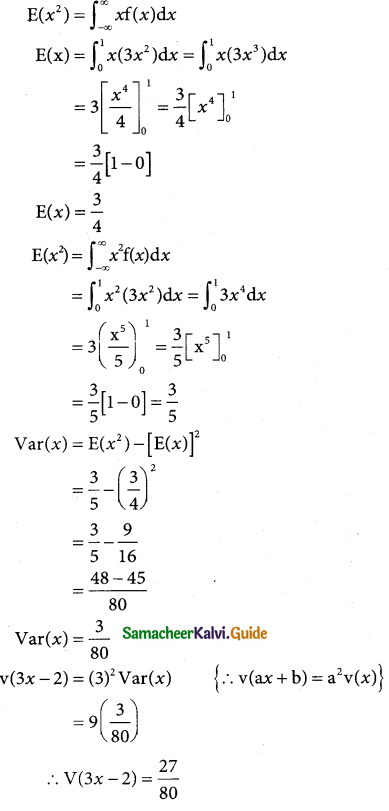Question 10.
The time to failure in thousands of hours of an important piece of electronic equipment used in a manufactured DVD player has the density function
f(x) = $$\left\{\begin{array}{l} 2 e^{-2 x}, x>0 \\ 0, \text { otherwise } \end{array}\right.$$
Find the expected life of this piece of equipment.
Solution:
Let X be the random variable